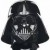# Which Test / Null Hypothesis to Use

Six Sigma – iSixSigma Forums Old Forums General Which Test / Null Hypothesis to Use

Viewing 5 posts - 1 through 5 (of 5 total)
• Author
Posts
• #45003

I want to test Silicone Products that are Post Cured at two different temperatures and then conduct tensile test of these samples**** Effect of Temperature on Tensile property of Material *************Sample Set 1 (30 samples): Set Point: 177 C Post cured for 2 hours Sample Set 2 (30 samples): Set Point: 166 C Post cured for 2 hours Aim: I need to evaluate the tensile test data and prove that the variation in temperature (post cured at 177 vs 166) has no significant effect on the tensile property of the product**** Effect of Time of Post Cure on Tensile property of Material *************Sample Set 2 (30 samples): Set Point: 177 C Post cured for 1 hour & 15 minSample Set 3 (30 samples): Set Point: 166 C Post cured for 1 hour & 15 minAim: I need to evaluate the tensile test data and prove that the variation in post curing time (post cured for 2 hours vs 1 hour & 15 min) has no significant effect on the tensile property of the productCan anyone please let me know which test to use  Should I use Independent Two-Sample t-Test  or ANOVA or some thing else.I am not able to write a Null hypothesis for this Please helpThanksRAHUL

0
#145614

So you are saying: 2 data sets, 1 input, 2 levels
Are both data sets normal?
If yes, then use an F-Test to determine if the variations are equal and do a 2-Sample T Test.
If no, then use a Levene’s Test to determine if the variations are equal, if they are equal (Ho>0.05) then use the Mann-Whitney Test, else you can use 2 Sample T or Mann-Whitney, but the results can’t be fully trusted.

0
#145616Ken Feldman
Participant

At first glance I would be tempted to do two t-tests assuming the tensile property is being measured as a continuous variable.
Ho: average of tensile at 177 for 2 hours = average of tensile at 166 for 2 hours
Ha:  averages are not equal
Ho: average of tensile at 177 for 75 min. = average of tensile at 166 for 75 min.
Ha: averages are not equal
Of course you could always do a 2 way ANOVA and get at both variables and possible interactions.

0
#145645

Darth,

I have to still conduct the tensile tests but I believe that the results will be that there is no significant difference on variation of temperature (177 v/s 166 ) or Time ( 2 hrs v/s 1 hour & 15 min)

Considering this if I use these Null hypotheses say

ONE
Ho: average of tensile at 177 for 2 hours = average of tensile at 166 for 2 hours
Ha:  averages are not equal

TWO
Ho: average of tensile at 177 for 2 hours = average of tensile at 177 for 1 hour and 15 min
Ha:  averages are not equal

And say after testing H0 is true How will I conclude my test

Because we cannot accept a Null hypothesis (it can be either rejected or Fail to be Rejected)

Is there a better way to soundly state that the average of tensile at 177 for 2 hours = average of tensile at 166 for 2 hours

Thanks
Rahul

0
#145649Ken Feldman
Participant

You have two variables, cure time and temperature.  You can look at four combinations
177 at 2 hours vs 166 at 2 hours and test for difference in temp at a given time
177 at 2 hours vs 177 at 75 min. and test for difference in time at given temp
177 at 75 min. vs 166 at 75 min. and again test for difference in temp at a given time
166 at 75 min vs 166 at 2 hrs. and again test for difference in time at a given temp.
Or do as I suggested and do a two way ANOVA so you can look at both variables and the possibility of an interaction between temp and time.  An interaction conclusion might say that for a temp of 177 use 75 min. but for a temp of 166 use 2 hours.
Don’t get too hung up on the wording of the null and alternate hypothesis.  Just know what the p value is telling you at the end.

0
Viewing 5 posts - 1 through 5 (of 5 total)

The forum ‘General’ is closed to new topics and replies.